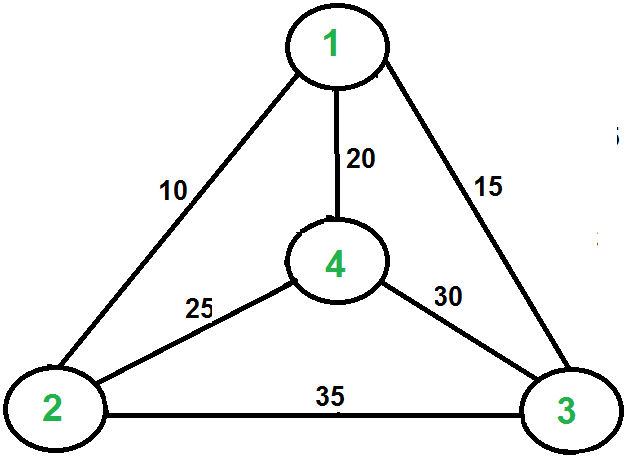# Travelling Salesman Problem | Greedy Approach

Given a 2D matrix tsp[][], where each row has the array of distances from that indexed city to all the other cities and -1 denotes that there doesn’t exist a path between those two indexed cities. The task is to print minimum cost in TSP cycle.

Examples:

Input:
tsp[][] = {{-1, 10, 15, 20},
{10, -1, 35, 25},
{15, 35, -1, 30},
{20, 25, 30, -1}};
Below is the given graph:Output: 80
Explanation:
We are trying to find out the path/route with the minimum cost such that our aim of visiting all cities once and return back to the source city is achieved. The path through which we can achieve that, can be represented as 1 -> 2 -> 4 -> 3 -> 1. Here, we started from city 1 and ended on the same visiting all other cities once on our way. The cost of our path/route is calculated as follows:
1 -> 2 = 10
2 -> 4 = 25
4 -> 3 = 30
3 -> 1 = 15
(All the costs are taken from the given 2D Array)
Hence, total cost = 10 + 25 + 30 + 15 = 80

Input:
tsp[][] = {{-1, 30, 25, 10},
{15, -1, 20, 40},
{10, 20, -1, 25},
{30, 10, 20, -1}};
Output: 50

## Recommended: Please try your approach on {IDE} first, before moving on to the solution.

We introduced Travelling Salesman Problem and discussed Naive and Dynamic Programming Solutions for the problem in the previous post. Both of the solutions are infeasible. In fact, there is no polynomial-time solution available for this problem as the problem is a known NP-Hard problem. There are approximate algorithms to solve the problem though.
This problem can be related to the Hamiltonian Cycle problem, in a way that here we know a Hamiltonian cycle exists in the graph, but our job is to find the cycle with minimum cost. Also, in a particular TSP graph, there can be many hamiltonian cycles but we need to output only one that satisfies our required aim of the problem.

Approach: This problem can be solved using Greedy Technique. Below are the steps:

1. Create two primary data holders:
• A list that holds the indices of the cities in terms of the input matrix of distances between cities.
• Result array which will have all cities that can be displayed out to the console in any manner.
2. Perform traversal on the given adjacency matrix tsp[][] for all the city and if the cost of the reaching any city from current city is less than current cost the update the cost.
3. Generate the minimum path cycle using the above step and return there minimum cost.

Below is the implementation of the above approach:

## Java

 `// Java program for the above approach ` `import` `java.util.*; ` ` `  `public` `class` `TSPGreedy { ` ` `  `    ``// Function to find the minimum ` `    ``// cost path for all the paths ` `    ``static` `void` `findMinRoute(``int``[][] tsp) ` `    ``{ ` `        ``int` `sum = ``0``; ` `        ``int` `counter = ``0``; ` `        ``int` `j = ``0``, i = ``0``; ` `        ``int` `min = Integer.MAX_VALUE; ` `        ``List visitedRouteList ` `            ``= ``new` `ArrayList<>(); ` ` `  `        ``// Starting from the 0th indexed ` `        ``// city i.e., the first city ` `        ``visitedRouteList.add(``0``); ` `        ``int``[] route = ``new` `int``[tsp.length]; ` ` `  `        ``// Traverse the adjacency ` `        ``// matrix tsp[][] ` `        ``while` `(i < tsp.length ` `               ``&& j < tsp[i].length) { ` ` `  `            ``// Corner of the Matrix ` `            ``if` `(counter >= tsp[i].length - ``1``) { ` `                ``break``; ` `            ``} ` ` `  `            ``// If this path is unvisited then ` `            ``// and if the cost is less then ` `            ``// update the cost ` `            ``if` `(j != i ` `                ``&& !(visitedRouteList.contains(j))) { ` `                ``if` `(tsp[i][j] < min) { ` `                    ``min = tsp[i][j]; ` `                    ``route[counter] = j + ``1``; ` `                ``} ` `            ``} ` `            ``j++; ` ` `  `            ``// Check all paths from the ` `            ``// ith indexed city ` `            ``if` `(j == tsp[i].length) { ` `                ``sum += min; ` `                ``min = Integer.MAX_VALUE; ` `                ``visitedRouteList.add(route[counter] - ``1``); ` `                ``j = ``0``; ` `                ``i = route[counter] - ``1``; ` `                ``counter++; ` `            ``} ` `        ``} ` ` `  `        ``// Update the ending city in array ` `        ``// from city which was last visited ` `        ``i = route[counter - ``1``] - ``1``; ` ` `  `        ``for` `(j = ``0``; j < tsp.length; j++) { ` ` `  `            ``if` `((i != j) && tsp[i][j] < min) { ` `                ``min = tsp[i][j]; ` `                ``route[counter] = j + ``1``; ` `            ``} ` `        ``} ` `        ``sum += min; ` ` `  `        ``// Started from the node where ` `        ``// we finished as well. ` `        ``System.out.print(``"Minimum Cost is : "``); ` `        ``System.out.println(sum); ` `    ``} ` ` `  `    ``// Driver Code ` `    ``public` `static` `void` `        ``main(String[] args) ` `    ``{ ` `        ``// Input Matrix ` `        ``int``[][] tsp = { ` `            ``{ -``1``, ``10``, ``15``, ``20` `}, ` `            ``{ ``10``, -``1``, ``35``, ``25` `}, ` `            ``{ ``15``, ``35``, -``1``, ``30` `}, ` `            ``{ ``20``, ``25``, ``30``, -``1` `} ` `        ``}; ` ` `  `        ``// Function Call ` `        ``findMinRoute(tsp); ` `    ``} ` `} `

Output:

```Minimum Cost is : 80
```

Time Complexity: O(N2*2N)
Auxiliary Space: O(N)My Personal Notes arrow_drop_upCheck out this Author's contributed articles.

If you like GeeksforGeeks and would like to contribute, you can also write an article using contribute.geeksforgeeks.org or mail your article to contribute@geeksforgeeks.org. See your article appearing on the GeeksforGeeks main page and help other Geeks.

Please Improve this article if you find anything incorrect by clicking on the "Improve Article" button below.